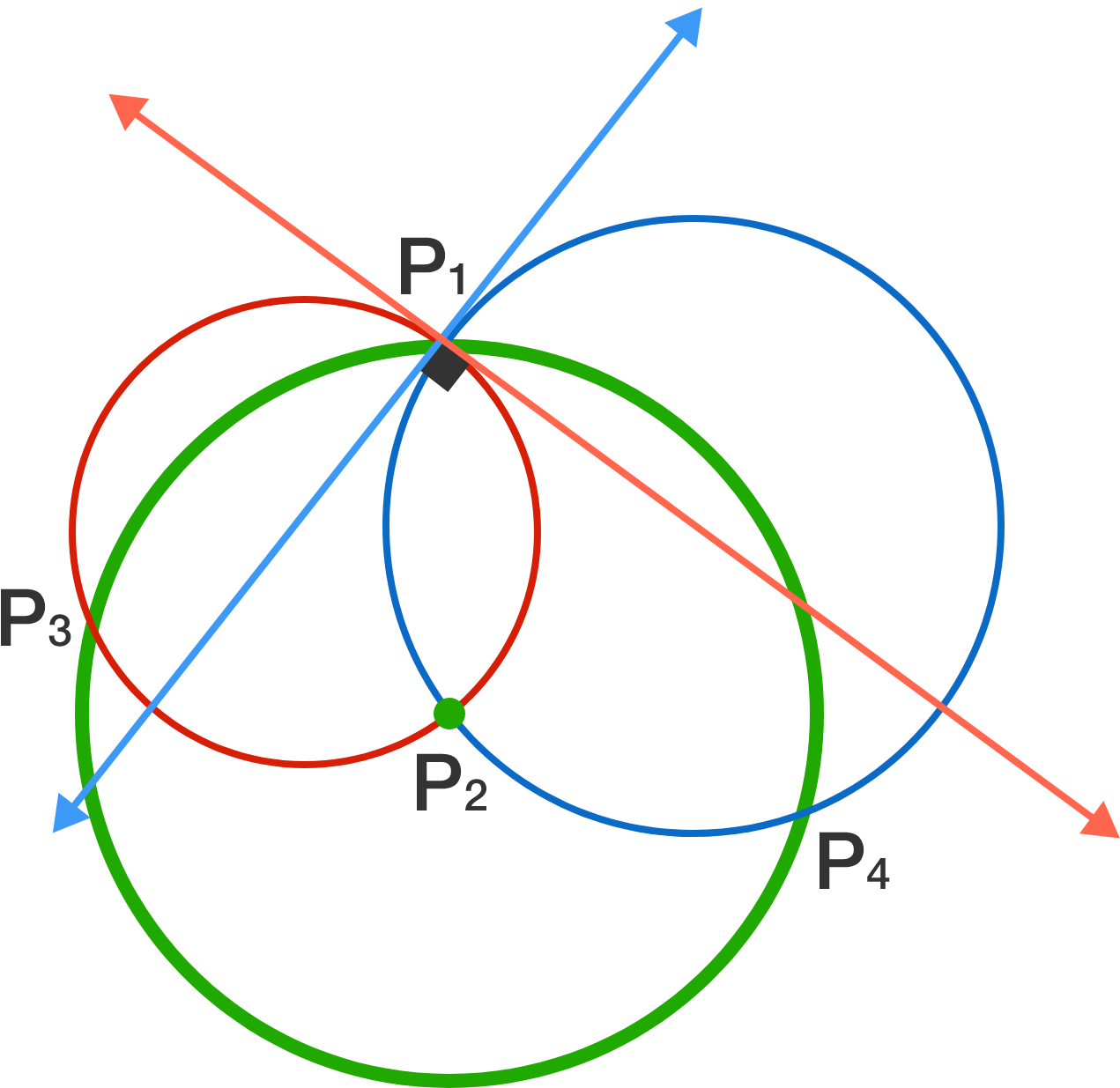# Circles, Tangents, And Triangles (Oh My...)

Geometry Level 5The red circle has radius 3 and the blue circle has radius 4. The tangents to one of their points of intersection, $P_1,$ are perpendicular.

The green circle is centered at the other point of intersection, $P_2,$ and passes through $P_1.$ It also intersects the red and blue circles at $P_3$ and $P_4,$ respectively.

What is the length of the altitude from $P_1$ in triangle $P_1P_3P_4?$

×